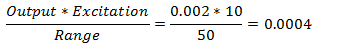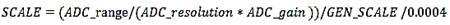﻿ Calculating SCALE values for load cells
 Required Parameters > The ANALOG group > ANALOG:SCALE

#### Calculating SCALE values for load cells

Many sensors produce an output in terms of units other than volts – in this case, an additional scaling factor must be applied to the scale calculation.  The scaling factor can be calculated once some basic information about the sensor is available.

In this example we will calculate the ANALOG:SCALE parameter for a typical load cell used to measure tension and compression so that we record the output in the same units that are used to calibrate the load cell.  The load cell data sheet provides the following information for this device:

Output                   2mV/V,

Excitation               10.0 VDC

The load cell output is specified in terms of volts output per volt of excitation at full load.  In this case, the manufacturer specifies a 10.0 Volt excitation voltage, so the load cell output will be 20mVat full load, which, for this load cell, is 50 pounds.  We now have enough information to calculate the sensor calibration factor:This sensor calibration factor can be using in the basic ANALOG:SCALE calculation to produce data values scaled directly in pounds:Assuming a GEN_SCALE value of 1.00, a 12-bit ADC (internal resolution of 4096) with an input range of ±10 volts, and a gain of x1, this produces an ANALOG:SCALE value of 12.207 that, at a quick glance, appears to be correct.  However the sensor output is, even at maximum load, very small and as a result, we have very poor resolution using this sensor and ADC combination.  The smallest change in tension or compression that can be recorded is one bit of ADC data – which, in this case, is about 12.2lbs.  In order to achieve a reasonable measurement resolution additional gain is required to amplify the output from the sensor to match the full ADC measurement range – this will, in turn, affect the ANALOG:SCALE parameter value.

Many modern ADC sampling devices can be programmed to use different input ranges by changing the ADC gain.  If we use an ADC_gain of x8 in the above scale calculations, we can improve the measurement resolution to about 1.5 lbs.  This resolution can be further improved by adding an additional gain stage in between the load cell and the ADC.